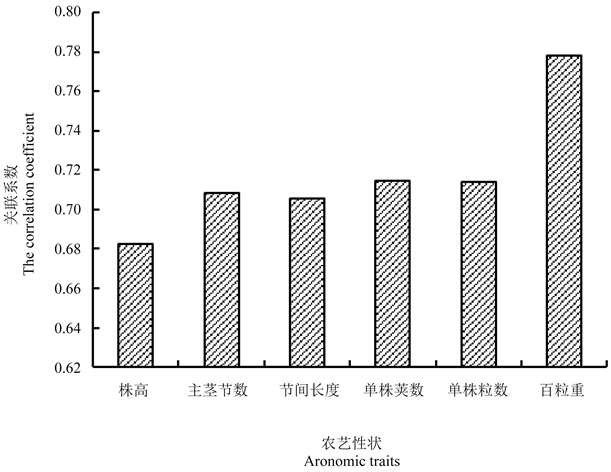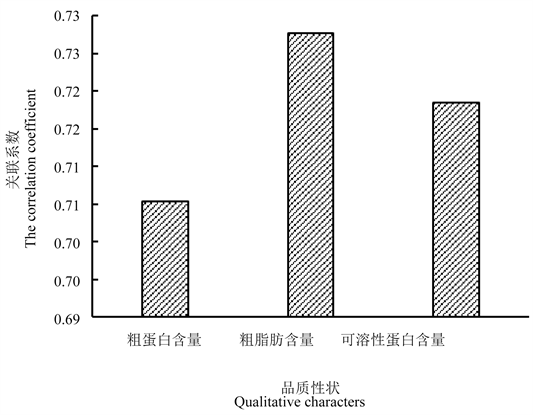﻿ 大豆主要农艺性状与品质灰色关联度分析

大豆主要农艺性状与品质灰色关联度分析Analysis of Grey Correlative Grades among the Main Agronomic Characters and Qualities of Soybean

Abstract: Using grey correlation analysis methods, the article evaluated the correlation between the main agronomic and quality characters of the soybean varieties and strains which were newly bred in Heilongjiang Province. The result showed that: there are high correlative grade among 100-seeds weight and quality characters. The correlative grade among plant height and quality characters was lower. The effect of economical character for oil content was lager.

1. 引言

2. 材料与方法

2.1. 试验材料

2.2. 试验设计

2.3. 分析方法

2.3.1. 数据标准化公式如下

${x}_{i}\left(k\right)=\frac{{{x}^{\prime }}_{i}\left(k\right)-{\stackrel{¯}{x}}_{i}}{{s}_{i}}$

2.3.2. 绝对差值计算公式如下

2.3.3. 相关系数计算公式如下

${L}_{oi}\left(k\right)=\frac{{\Delta }_{\mathrm{min}}+\rho {\Delta }_{\mathrm{max}}}{\Delta i\left(k\right)+\rho {\Delta }_{\mathrm{max}}}$

2.3.4. 关联度的计算公式如下

${r}_{oi}=\frac{1}{N}\underset{K=1}{\overset{N}{\sum }}{L}_{oi}\left( k \right)$

N代表材料个数N = 600。

3. 结果与分析

3.1. 原始数据无钢化处理Table 1. Information of major agronomic characters of soybean after data processing

3.2. 关联系数和关联度Table 2. Grey correlative grades among agronomic characters and qualitative character of soybeanFigure 1. Grey correlative grades among agronomic characters and qualityFigure 2. Grey correlative grades among agronomic traits and the contents of crude protein, crude fat and soluble protein

4. 结论

“政府间国际科技创新合作”重点专项(2019YFE0105900)。

NOTES

*通讯作者。

 杨庆凯, 论大豆蛋白质与油分含量品质的变化及影响的因素[J]. 大豆科学, 2000, 19(4): 386-391.

 徐淑霞, 周青, 杨慧凤. 利用灰色关联度分析大豆新品系[J]. 农业科技通讯, 2015(4): 94-96.

 郭瑞林. 作物灰色育种学[M]. 北京: 中国农业科技出版社, 1995: 3-4.

 童燕, 肖敬德, 张光. 大豆主要农艺性状与品质性状间的灰色关联度分析[J]. 大豆科技, 2013(2): 26-29.

 胡振邦, 朱荣盛, 高运来. 黑龙江省大豆品种单株粒重与其他农艺性状的灰色关联度分析[J]. 东北农业大学学报, 2011, 42(11): 57-62.

 张君, 王丕武. 大豆主要性状间的灰色关联度分析[J]. 沈阳农业大学学报, 2004, 35(1): 1-3.

Top